# Class 10th Maths NCERT Solutions for Chapter 15 - Probability(Exercise 15.1)

## NCERT Solutions for Chapter 15 Exercise 15.2

In this page we have NCERT solutions for Class 10 Maths Chapter 15: probability for EXERCISE 15.1 on Pages 308,309,310 . Hope you like them and do not forget to like , social_share and comment at the end of the page.
Question 1
Complete the following statements:
1. Probability of an event E + Probability of the event ‘not E’ =…………….
2. The probability of an event that cannot happen is ……. Such an event is called ……..
3. The probability of an event that is certain to happen is……….     . Such an event is called …….
4. The sum of the probabilities of all the elementary events of an experiment is ………..
5. The probability of an event is greater than or equal to ……….and less than or equal to ………
Solution
1. We know that
The event $\bar A$, representing ‘not A’, is called the complement of the event A. We also say that $\bar A$ and A are complementary events.
Also $P(A) +P(\bar A)=1$
2. The probability of an event (U) which is impossible to occur is 0. Such an event is called an impossible event
$P(U)=0$
3. The probability of an event ( X) which is sure (or certain) to occur is 1. Such an event is called a sure event or a certain event
$P(X)=1$
4. An event having only one outcome of the experiment is called an elementary event.
Also The sum of the probabilities of all the elementary events of an experiment is 1.
i.e. If we three elementary event A,B,C in  the experiment ,then
$P(A)+P(B) +P(C)=1$
5. Probability of any event can be as
$$0 \le P(E) \le 1$$
Question 2
Which of the following experiments have equally likely outcomes? Explain.
1. A driver attempts to start a car. The car starts or does not start.
2. A player attempts to shoot a basketball. She/he shoots or misses the shot.
4. A baby is born. It is a boy or a girl
Solution
1. Not equally likely
2. Not equally likely
3. Equally likely as both have equal possibility
4. Equally likely as the baby born has equal possibility of boy or girl
Question 3
Why is tossing a coin considered to be a fair way of deciding which team should get the ball at the beginning of a football game?
Solution
Since the possibility of getting head and tail are equally likely. It will be unbiased and unpredictable
Question 4
Which of the following cannot be the probability of the event
1. 2/3
2. -1.5
3. 15%
4. .7

Solution
(b) as probability cannot be negative

Question 5
if P(E) = 0.05, what is the probability  of ‘Not E’?
Solution
The event $\bar E$, representing ‘not E’, is called the complement of the event E. We also say that $\bar E$ and E are complementary events. Also
$P(E) +P(\bar E)=1$
Here P(E) =.05
So $P(E) +P(\bar E)=1$
$P(\bar E)=1-.05=.95$
Question 6
A bag contains lemon colored candies. Malini takes out one candy without looking into the bag. What is the probability that she takes out
1. An orange colored candy
2. An lemon colored candy

Solution
The bag does not contain any orange candy
1. So probability of getting orange candy is zero
2. Since she will always get lemon candy, probability is 1

Question 7
It is given that in a group of 3 students, the probability of 2 students not having the same birthday is .992.   What is the probability that 2 students have the same birthday?
Solution
Let A be the event 2 students not having the same birthday And B be the event 2 students having same birthday
These both the events are complimentary so
P(A)+P(B)=1
P(B)=1-.992=.008
Question 8
A bag contains 3 red balls and 5 black balls. A ball is drawn at random from the bag.  What is the probability that the ball drawn is
1. Red
2. No red
Solution:
Total number of balls in the bad n(B)=5+3=8
No of ball which are red =3
1. Let A be event of getting red ball Probability of getting red ball P(A)= No of red balls/Total number of balls= 3/8
2. Let B be the event of getting non red ball P(B) =No of black balls/ Total number of  balls=5/8
Question 9
A box contains 5 red marbles, 8 white marbles and 4 green marbles. One marble is taken out of the box at random. What is the probability that the marble taken out will be?
(i) red?
(ii) white?
(iii) not green?

Given
No. of red marbles(r) = 5
No. of white marbles (w)=8
No. of green marbles(g) = 4
Total no. of balls(n) = 5+8+4 = 17

(i) Number of Favorable outcome to event (Red marble) =r= 5
Probability of taking out red marble =r/n= 5/17

(ii) Number of Favorable outcome to event (white marble) =w= 8
Probability of taking out red marble = w/n=8/17

(iii) Number of Favorable outcome to events (no green) = r+w=13
Probability of taking out (not green) = 13/17

Question 10
A piggy bank contains hundred 50p coins, fifty Rs1 coins, twenty Rs2 coins and ten Rs5 coins. If it is equally likely that one of the coins will fall out when the bank is turned upside down, what is the probability that the coin
(i) will be a 50 p coin?
(ii) will not be a Rs5 coin?

Given
No. of 50p coins = 100
No. of Rs1 coins = 50
No. of Rs2 coins = 20
No. of Rs5 coins = 10
Total no. of coins = 100 + 50 + 20 + 10 = 180

(i) Number of Favorable outcome to event (50p coin) = 100
Probability that it will be 50p coins = 100/180 = 5/9

(ii) Number of Favorable outcome to event (not Rs 5 coin) =All the coins except Rs5 coin= 100+50+20 = 170
Probability that it will be 50p coins = 170/180 = 17/18

Question 11
Gopi buys a fish from a shop for his aquarium. The shopkeeper takes out one fish at random from a tank containing 5 male fish and 8 female fish (see Fig. 15.4). What is the probability that the fish taken out is a male fish?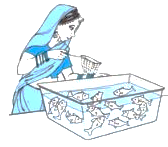Given
No. of male fish in the tank(m) = 5
no. of female fish in the tank(f) = 8
Total number of fish in the tank (n)= 5 + 8 = 13
Then Number of Favorable outcome to event (male fish) =m= 5
Probability of taking out a male fish =m/n= 5/13

Question 12
A game of chance consists of spinning an arrow which comes to rest pointing at one of the numbers 1, 2, 3, 4, 5, 6, 7, 8 (see below figure), and these are equally likely outcomes. What is the probability that it will point at
(i) 8?
(ii) an odd number?
(iii) a number greater than 2?
(iv) a number less than 9?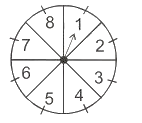Total Possible no. of events(n) = 8
(i) Number of Favorable outcome to  event (8)= 1
Probability that it will point at 8 = 1/8

(ii) Odd numbers = 1, 3, 5 and 7
Number of Favorable outcome to  event (odd number)= 4
Probability that it will be an odd number = 4/8 = 1/2

(iii) Numbers greater than 2 will be 3, 4, 5, 6, 7 and 8
So Number of Favorable outcome to  event (Numbers greater than 2)= 6
Probability that a number greater than 2 = 6/8 = 3/4

(iv) Numbers less than 9 = 1,2,3,4,5,6,7,8
Number of Favorable outcome to  event (Numbers less than 9)= = 8
Probability that a number less than 9 = 8/8 = 1
So it is a sure event

Question 13
A die is thrown once. Find the probability of getting
(i) a prime number;
(ii) a number lying between 2 and 6;
(iii) an odd number.

Possible numbers of events on throwing a dice(n) = 6
Numbers on dice = 1,2,3,4,5 and 6

(i) Now Prime numbers = 2, 3 and 5
So   Number of Favorable outcome to  event (prime number)= 3
Probability that it will be a prime number = 3/6 = 1/2

(ii) Now Numbers lying between 2 and 6 = 3, 4 and 5
So Number of Favorable outcome to  event (Numbers lying between 2 and 6) = 3
Probability that a number between 2 and 6 = 3/6 = 1/2

(iii) Now Odd numbers = 1, 3 and 5
Number of Favorable outcome to  event (odd numbers)  = 3
Probability that it will be an odd number = 3/6 = 1/2

Question 14
One card is drawn from a well-shuffled deck of 52 cards. Find the probability of getting
(i) a king of red colour
(ii) a face card
(iii) a red face card
(iv) the jack of hearts
(vi) the queen of diamonds

Possible numbers of events = 52

(i) Numbers of king of red colour = 2
Probability of getting a king of red colour = 2/52 = 1/26

(ii) Numbers of face cards = 12
Probability of getting a face card = 12/52 = 3/13

(iii) Numbers of red face cards = 6
Probability of getting a king of red colour = 6/52 = 3/26

(iv) Numbers of jack of hearts =1
Probability of getting a king of red colour = 1/526

(v) Numbers of king of spade = 13
Probability of getting a king of red colour = 13/52 = 1/4

(vi) Numbers of queen of diamonds = 1
Probability of getting a king of red colour = 1/52

Question 15
Five cards the ten, jack, queen, king and ace of diamonds, are well-shuffled with their face downwards. One card is then picked up at random.
(i) What is the probability that the card is the queen?
(ii) If the queen is drawn and put aside, what is the probability that the second card picked up is (a) an ace? (b) a queen?

Total numbers of cards = 5

(i) Numbers of queen = 1
Probability of picking a queen = 1/5

(ii) When queen is drawn and put aside then total numbers of cards left is 4
(a) Numbers of ace = 1
Probability of picking an ace = 1/4
(a) Numbers of queen = 0
Probability of picking a queen = 0/4 = 0

Question 16
12 defective pens are accidentally mixed with 132 good ones. It is not possible to just look at a pen and tell whether or not it is defective. One pen is taken out at random from this lot. Determine the probability that the pen taken out is a good one.

Given
Numbers of defective pens(d) = 12
Numbers of good pens(g) = 132
Total numbers of pen =d+g= 132 + 12 = 144 pens
Number of Favorable outcome to event (good pen) = 132
Probability of getting a good pen = g/(d+g)=132/144 = 11/12

Question 17
(i) A lot of 20 bulbs contain 4 defective ones. One bulb is drawn at random from the lot. What is the probability that this bulb is defective?
(ii) Suppose the bulb drawn in (i) is not defective and is not replaced. Now one bulb is drawn at random from the rest. What is the probability that this bulb is not defective?

(i) Total numbers of bulbs = 20
Numbers of defective bulbs = 4
Probability of getting a defective bulb = 4/20 = 1/5

(ii) One non defective bulb is drawn in (i) then the total numbers of bulb left is 19
Total numbers of events = 19
Favorable numbers of events = 19 - 4 = 15
Probability that the bulb is not defective = 15/19

Question 18
A box contains 90 discs which are numbered from 1 to 90. If one disc is drawn at random from the box, find the probability that it bears
(i) a two-digit number
(ii) a perfect square number
(iii) a number divisible by 5.

Total numbers of discs = 90

(i) Number of Favorable outcome to  event (two digit number) = 81
Probability that it bears a two-digit number = 81/90 = 9/10

(ii) Perfect square numbers are  1, 4, 9, 16, 25, 36, 49, 64 and 81
So Number of Favorable outcome to  event (Perfect square numbers )= 9
Probability of getting a perfect square number = 9/90 = 1/10

(iii) Numbers which are divisible by 5 are  5, 10, 15, 20, 25, 30, 35, 40, 45, 50, 55, 60, 65, 70, 75, 80, 85 and 90
So Number of Favorable outcome to  event (Numbers which are divisible by 5 )= 18
Probability of getting a number divisible by 5 = 18/90 = 1/5

Question 19
A child has a die whose six faces show the letters as given below: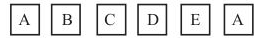The die is thrown once. What is the probability of getting (i) A? (ii) D?

Total numbers of events = 6

(i) Total numbers of faces having A on it = 2
Probability of getting A = 2/6 = 1/3

(ii) Total numbers of faces having D on it = 1
Probability of getting A = 1/6

Question 20
Suppose you drop a die at random on the rectangular region shown in Fig. 15.6. What is the probability that it will land inside the circle with diameter 1m?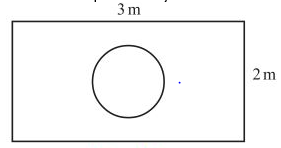Area of the rectangle = $(3 \times 2) \; m^2= 6 \; m^2$
Area of the circle = $\pi r^2= \pi (\frac {1}{2})^2 \; m^2 = \frac {\pi}{4} \; m^2 Probability that die will land inside the circle =$ \frac {\frac {\pi}{4}}{6} = \frac {\pi}{24}$Question 21 A lot consists of 144 ball pens of which 20 are defective and the others are good. Nuri will buy a pen if it is good, but will not buy if it is defective. The shopkeeper draws one pen at random and gives it to her. What is the probability that? (i) She will buy it? (ii) She will not buy it? Answer Total numbers of pens = 144 Numbers of defective pens = 20 Numbers of non defective pens = 144 - 20 = 124 (i) Numbers of favorable events = 124 Probability that she will buy it =$\frac {124}{144} = \frac {31}{36}$(ii) Numbers of favorable events = 20 Probability that she will not buy it =$ \frac {20}{144} = \frac {5}{36}$Question 22. Two dice, one blue and one grey, are thrown at the same time. Complete the following table: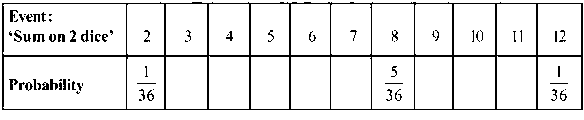(ii) A student argues that ‘there are 11 possible outcomes 2, 3, 4, 5, 6, 7, 8, 9, 10, 11 and 12. Therefore, each of them has a probability 1/11. Do you agree with this argument? Justify your answer. Answer Events that can happen on throwing two dices are: (1, 1), (1, 2), (1, 3), (1, 4), (1, 5), (1, 6) (2, 1), (2, 2), (2, 3), (2, 4), (2, 5), (2, 6) (3, 1), (3, 2), (3, 3), (3, 4), (3, 5), (3, 6) (4, 1), (4, 2), (4, 3), (4, 4), (4, 5), (4, 6) (5, 1), (5, 2), (5, 3), (5, 4), (5, 5), (5, 6) (6, 1), (6, 2), (6, 3), (6, 4), (6, 5), (6, 6) Total numbers of events : 6 × 6 = 36 (i) To get sum as 2, possible outcomes = (1,1) To get sum as 3, possible outcomes = (1,2) and (2,1) To get sum as 4, possible outcomes = (1,3); (3,1); and (2,2) To get sum as 5, possible outcomes = (1,4); (4,1); (2,3); and (3,2) To get sum as 6, possible outcomes = (1,5); (5,1); (2,4); (4,2); and (3,3) To get sum as 7, possible outcomes = (1,6); (6,1); (5,2); (2,5); (4,3); and (3,4) To get sum as 8, possible outcomes = (2,6); (6,2); (3,5); (5,3); and (4,4) To get sum as 9, possible outcomes = (3,6); (6,3); (4,5); and (5,4) To get sum as 10, possible outcomes = (4,6); (6,4) and (5,5) To get sum as 11, possible outcomes = (5,6) and (6,5) To get sum as 12, possible outcomes = (6,6)  Event: Sum on 2 dice 2 3 4 5 6 7 8 9 10 11 12 Probability 1/36 2/36 3/36 4/36 5/36 6/36 5/36 4/36 3/36 2/36 1/36 (ii) No, i don't agree with the argument. It is already justified in (i). Question 23 A game consists of tossing a one-rupee coin 3 times and noting its outcome each time. Hanif wins if all the tosses give the same result i.e., three heads or three tails, and loses otherwise. Calculate the probability that Hanif will lose the game. Answer Events that can happen in tossing 3 coins = HHH, HHT, HTH, THH, TTH, HTT, THT, TTT Total number of events = 8 Hint if will lose the game if he gets HHT, HTH, THH, TTH, HTT, THT Favorable number of elementary events = 6 Probability of losing the game =$\frac {6}{8} = \frac {3}{4}$Question 24 A die is thrown twice. What is the probability that (i) 5 will not come up either time? (ii) 5 will come up at least once? [Hint: Throwing a die twice and throwing two dice simultaneously are treated as the same experiment] Answer (i) Total possible outcome= 36 Number of favorable outcome when 5 comes at least once = 11 So Number of favorable outcome when 5 will not come up = 36-11=25 Required probability =$\frac {25}{36}$(ii) Number of favorable outcome when 5 comes at least once = 11 Probability =$\frac {11}{36}$Question 25 Which of the following arguments are correct and which are not correct? Give reasons for your answer. (i) If two coins are tossed simultaneously there are three possible outcomes—two heads, two tails or one of each. Therefore, for each of these outcomes, the probability is 1/3 (ii) If a die is thrown, there are two possible outcomes—an odd number or an even number. Therefore, the probability of getting an odd number is 1/2 Answer (i) The statement is incorrect Possible events = (H, H); (H, T); (T, H) and (T, T) Probability of getting two heads =$\frac {1}{4}$Probability of getting one of the each =$\frac {2}{4} = \frac {1}{2}$(ii) Correct. The two outcomes considered are equally likely. link to this page by copying the following text Also ReadGo back to Class 10 Main Page using below links ### Practice Question Question 1 What is$1 - \sqrt {3}\$ ?
A) Non terminating repeating
B) Non terminating non repeating
C) Terminating
D) None of the above
Question 2 The volume of the largest right circular cone that can be cut out from a cube of edge 4.2 cm is?
A) 19.4 cm3
B) 12 cm3
C) 78.6 cm3
D) 58.2 cm3
Question 3 The sum of the first three terms of an AP is 33. If the product of the first and the third term exceeds the second term by 29, the AP is ?
A) 2 ,21,11
B) 1,10,19
C) -1 ,8,17
D) 2 ,11,20